After my post on detecting outliers in multivariate data in SAS by using the MCD method, Peter Flom commented "when there are a bunch of dimensions, every data point is an outlier" and remarked on the curse of dimensionality. What he meant is that most points in a high-dimensional cloud of points are far away from the center of the cloud.

### Distances and outliers in high dimensions

You can demonstrate this fact with a simulation. Suppose that you simulate 1,000 observations from a multivariate normal distribution (denoted MVN(0,Σ)) in d-dimensional space. Because the density of the distribution is highest near the mean (in this case, the origin), most points are "close" to the mean. But how close is "close"? You might extrapolate from your knowledge of the univariate normal distribution and try to define an "outlier" to be any point whose distance from the origin is more than some constant, such as five standardized units.

That sounds good, right? In one dimension, an observation from a normal distribution that is more than 5 standard deviations away from the mean is an extreme outlier. Let's see what happens for high-dimensional data. The following SAS/IML program does the following:

1. Simulates a random sample from the MVN(0,Σ) distribution.
2. Uses the Mahalanobis module to compute the Mahalanobis distance between each point and the origin. The Mahalanobis distance is a standardized distance that takes into account correlations between the variables.
3. Computes the distance of the closest point to the origin.

```proc iml; /* Helper function: return correlation matrix with "compound symmetry" structure: {v+v1 v1 v1, v1 v+v1 v1, v1 v1 v+v1 }; */ start CompSym(N, v, v1); return( j(N,N,v1) + diag( j(N,1,v) ) ); finish; load module=Mahalanobis; /* or insert definition of module here */   call randseed(12345); N = 1000; /* sample size */ rho = 0.6; /* rho = corr(x_i, x_j) for i^=j */ dim = T(do(5,200,5)); /* dim=5,10,15,...,200 */ MinDist = j(nrow(dim),1); /* minimum distance to center */ do i = 1 to nrow(dim); d = dim[i]; mu = j(d,1,0); Sigma = CompSym(d,1-rho,rho); /* get (d x d) correlation matrix */ X = randnormal(N, mu, Sigma); /* X ~ MVN(mu, Sigma) */ dist = Mahalanobis(X, mu, Sigma); MinDist[i] = min(dist); /* minimum distance to mu */ end;```

The following graph shows the distance of the closest point to the origin for various dimensions.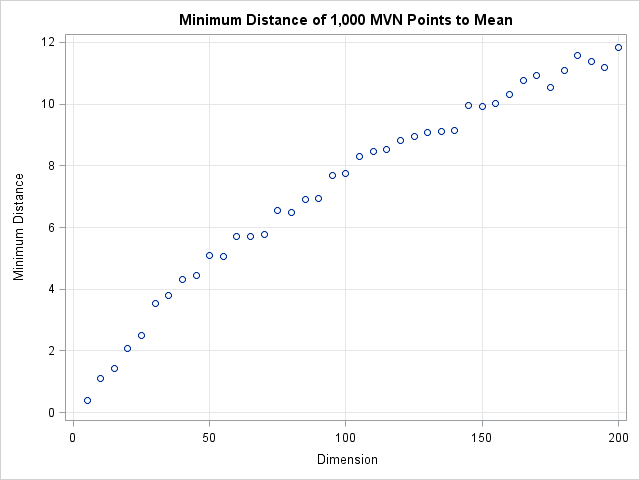The graph shows that the minimum distance to the origin is a function of the dimension. In 50 dimensions, every point of the multivariate normal distribution is more than 5 standardized units away from the origin. In 150 dimensions, every point is more than 10 standardized units away! Consequently, you cannot define outliers a priori to be observations that are more than 5 units away from the mean. If you do, you will, as Peter said, conclude that in 50 dimensions every point is an outlier.

### How to define an outlier cutoff in high dimensions

The resolution to this dilemma is to incorporate the number of dimensions into the definition of a cutoff value. For multivariate normal data in d dimensions, you can show that the squared Mahalanobis distances are distributed like a chi-square distribution with d degrees of freedom. (This is discussed in the article "Testing data for multivariate normality.") Therefore, you can use quantiles of the chi-square distribution to define outliers. A standard technique (which is used by the ROBUSTREG procedure to classify outliers) is to define an outlier to be an observation whose distance to the mean exceeds the 97.5th percentile. The following graph shows a the 97.5th percentile as a function of the dimension d. The graph shows that the cutoff distance is greater than the minimum distance and that the two distances increase in tandem.

`cutoff = sqrt(quantile("chisquare", 0.975, dim)); /* 97.5th pctl as function of dimension */`If you use the chi-square cutoff values, about 2.5% of the observations will be classified as outliers when the data is truly multivariate normal. (If the data are contaminated by some other distribution, the percentage could be higher.) You can add a few lines to the previous program in order to compute the percentage of outliers when this chi-square criterion is used:

```/* put outside DO loop */ PctOutliers = j(nrow(dim),1);/* pct outliers for chi-square d cutoff */ ... /* put inside DO loop */ cutoff = sqrt( quantile("chisquare", 0.975, d) ); /* dist^2 ~ chi-square */ PctOutliers[i] = sum(dist>cutoff)/N; /* dist > statistical cutoff */ ...```

The following graph shows the percentage of simulated observations that are classified as outliers when you use this scheme. Notice that the percentage of outliers is close to 2.5% independent of the dimension of the problem! By knowing the distribution of distances in high-dimensional MVN data, we are able to define a cutoff value that does not classify every point as an outlier.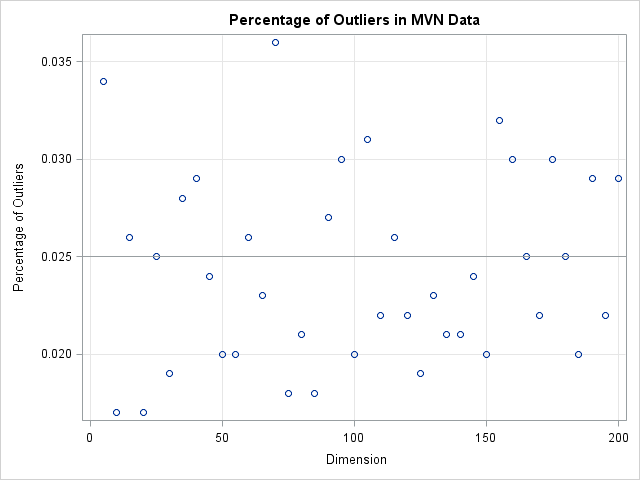To conclude, in high dimensions every data point is far away from the mean. If you use a constant cutoff value then you will erroneously classify every data point as an outlier. However, you can use statistical theory to define a reliable rule to detect outliers, regardless of the dimension of the data.A recent discussion on the SAS-L discussion forum concerned how to implement linear interpolation in SAS. Some people suggested using PROC EXPAND in SAS/ETS software, whereas others proposed a DATA step solution.

For me, the SAS/IML language provides a natural programming environment to implement an interpolation scheme. It also provides the flexibility to handle missing values and values that are outside of the range of the data. In this article I'll describe how to implement a simple interpolation scheme and how to modify the simple scheme to support missing values and other complications.

### What is interpolation?

There are many kinds of interpolation, but when most people use the term "interpolation" they are talking about linear interpolation. Given two points (x1,y1) and (x2,y2) and a value x such that x is in the interval [x1, x2], linear interpolation uses the point-slope formula from high-school algebra to compute a value of y such that (x,y) is on the line segment between (x1,y1) and (x2,y2). Linear interpolation between two points can be handled by defining the following SAS/IML module:

```proc iml; /* Interpolate such that (x,y) is on line segment between (x1,y1) and (x2,y2) */ start LinInterpPt(x1, x2, y1, y2, x); m = (y2-y1)/(x2-x1); /* slope */ return( m#(x-x1) + y1 ); /* point-slope formula */ finish;```

The following graph shows a linear interpolation scheme. The data are shown with round markers. The line segments are the graph of the piecewise-linear interpolation function for the data. Several points (shown with star-shaped markers) are interpolated. The next section shows how to compute the interpolated values.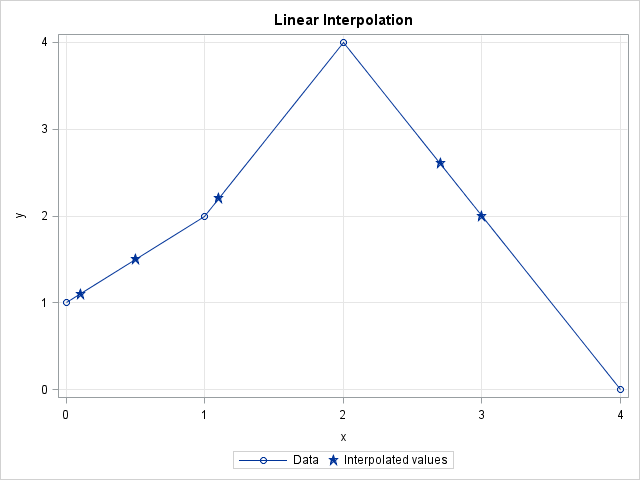### Linear interpolation: A simple function

Suppose you have a set of points (x1,y1), (x2,y2), ..., (xn,yn) that are ordered so that x1 < x2 < ... < xn. It is not difficult to use the LinearInterpPt function to interpolate a value, v in the interval [x1, xn): you simply find the value of k so that xk ≤ v < xk+1 and then call the LinInterpPt function to interpolate. If you have more than one value to interpolate, you can loop over the values, as shown in the following function:

```start Last(x); /* Helper module: return last element in a vector */ return( x[nrow(x)*ncol(x)] ); finish;   /* Linear interpolation: simple version */ start LinInterp1(x, y, v); /* Given column vectors (x, y), interpolate values for column vector v. Assume: 1. no missing values in x, y, or v 2. the values of x are unique and sorted 3. each element of v is in the interval [minX, maxX) */ fv = j(nrow(v),1); /* allocate return vector */ do i = 1 to nrow(v); k = Last( loc(x <= v[i] )); /* largest x less than v[i] */ fv[i] = LinInterpPt(x[k], x[k+1], y[k], y[k+1], v[i]); end; return( fv ); finish;   /* test it on some data */ xy = {0 1, 1 2, 2 4, 4 0 }; v = {0.1, 1.1, 0.5, 2.7, 3}; /* interpolate at these points */ fv = LinInterp1(xy[,1], xy[,2], v); print v fv;```The elements of v and fv are plotted as stars on the graph in the preceding section. The round markers are the data in the xy matrix.

### Linear interpolation: A more robust implementation

The LinearInterp1 function is short, simple, and does the job, but it makes a lot of assumptions about the structure of the (x,y) data and the values to be interpolated. In practice, it is more useful to have a function that makes fewer assumptions and also handles various error conditions. In particular, a useful function would do the following:

1. Handle the case where an element of v equals the maximum value xn.
2. Handle missing values in x or y by deleting those ordered pairs from the set of data values.
3. Check that the nonmissing values of x are unique and issue an error message if they are not. (Alternatively, you could combine repeated values by using the mean or median of the y values.)
4. Sort the ordered pairs by x.
5. Handle elements of v that are outside of interval [x1, xn]. The simplest scheme is to return missing values for these elements. I do not recommend extrapolation.
The first case is easily handled in the LinearInterp module. To implement the other cases, I'll write a module called Interp that handles cases 2–5 and then passes the remaining values of x, y, and v to the LinearInterp module. The following modules implement this strategy:
```start LinInterp(x, y, v); /* Assume x and v are sorted and x is unique. Return f(v; x,y) where f is linear interpolation function determined by (x,y) */ fv = j(nrow(v),1); /* allocate return vector */ begin = 1; /* set default value */ idx = loc(v=x); /* 1. handle v[i]=xMin separately */ if ncol(idx)>0 then do; fv[idx] = y; begin = Last(idx) + 1; end; do i = begin to nrow(v); /* remaining values have xMin < v[i] <= xMax */ k = Last( loc(x < v[i] )); /* largest x less than v[i] */ fv[i] = LinInterpPt(x[k], x[k+1], y[k], y[k+1], v[i]); end; return( fv ); finish;   start Interp(_x, _y, _v); /* Given column vectors (x, y), return interpolated values of column vector v */ /* 2. Delete missing values in x and y */ idx = loc( _x^=. & _y^=. ); if ncol(idx)<2 then return( j(nrow(_v),1,.) ); x = _x[idx]; y = _y[idx];   /* 3. check that the nonmissing values of x are unique */ u = unique(x); if nrow(x)^=ncol(u) then do; print "ERROR: x cannot contain duplicate values"; stop; /* Alternatively, use mean or median to handle repeated values */ end;   /* 4. sort by x */ xy = x || y; call sort(xy, 1); x = xy[,1]; y = xy[,2];   /* 5. don't interpolate outside of [minX, maxX]; delete missing values in v */ minX = x; maxX = Last(x); interpIdx = loc( _v>=minX & _v<=maxX & _v^=. ); if ncol(interpIdx)=0 then return( j(nrow(_v),1,.) );   /* set up return vector; return missing for v out of range */ fv = j(nrow(_v),1,.); fv[interpIdx] = LinInterp(x, y, _v[interpIdx] ); return (fv); finish;   /* test the modules */ xy = {0 1, . 3, 2 4, 4 0, 5 ., 1 2}; v = {0.1, 1.1, 0.5, 2.7, 3, ., 4, 5, -1}; /* interpolate at these points */ fv = Interp(xy[,1], xy[,2], v); print v fv;```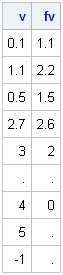The first five values of v are the same as in the previous section. The last four elements are values that would cause errors in the simple module.

Clearly it takes more work to handle missing values, unordered data, and out-of-range conditions. The simple function with six statements has evolved into two functions with about 30 statements. This is a classic example of "the programmer's 80-20 rule": In a typical program, only 20% of the statements are needed to compute the results; the other 80% handle errors and degenerate situations.

In spite of the extra work, the end result is worth it. I now have a robust module that performs linear interpolation for a wide variety of input data and interpolation values.Most statistical programmers have seen a graph of a normal distribution that approximates a binomial distribution. The figure is often accompanied by a statement that gives guidelines for when the approximation is valid. For example, if the binomial distribution describes an experiment with n trials and the probability of success for each trial is p, then the quantity np(1-p) must be larger than some cutoff (often 5 is used, but sometimes 10) in order for the approximation to be valid.

The following SAS/IML statements compute the binomial probabilities for n=50 and p=0.8 (so that np(1-p)=8) and for the approximating normal curve, which has mean np and variance np(1-p). These values are plotted by the SGPLOT procedure (SGPLOT statements not shown):

```proc iml; n = 50; /* number of trials: better approx for 100, 200, etc. */ p = 0.8; /* probability of success for each trial */ mu = n*p;/* classic approximation of binomial pdf by normal pdf */ stddev = sqrt(n*p*(1-p)); xMin = mu - 4*stddev; xMax = mu+4*stddev; x1 = T(floor(xMin):ceil(xMax)); /* evaluate binomial at integers */ y1 = pdf("Binom", x1, p, n); x2 = T(do(xMin, xMax, (xMax-xMin)/101)); y2 = pdf("Normal", x2, mu, stddev); /* write values to SAS data set; plot with SGPLOT */```The approximation looks good. However, the figure suggests that you need to be careful if you use a normal distribution to approximate extreme quantiles (such as 0.01 or 0.99) of the binomial distribution. Although the "middles" of the two distributions agree well, there appears to be les agreement in the tails. Also, recall that the quantiles of a discrete distribution are integers, which provides yet another source of error when approximating a binomial quantile with a normal quantile. This is evident when you overlay the normal CDF on the binomial CDF, as in the following figure:

```prob = T( do(0.01, 0.99, 0.005) ); q = quantile("binom", prob, p, n); /* ALWAYS an integer! */ qNormal = quantile("normal", prob, mu, stddev); diff = q - qNormal; /* error from approximating binomial quantiles */ /* write values to SAS data set; plot with SGPLOT */```The graph shows that there is considerable error for quantiles near zero and one. Is this important? It can be. For example, if you are creating a funnel plot for proportions the curves on the funnel plot are computed with the quantiles 0.001, 0.025, 0.975, and 0.999. These are extreme quantiles, but they are used to compute the funnel-like control limits, which are the most important feature of the plot. I suspect this is why David Spiegelhalter in his paper "Funnel plots for comparing institutional performance" used a rather complicated formula (in Appendix A.1.1) to interpolate the binomial quantiles in the funnel plot for proportions, rather than using a binomial approximation.

The following graph shows a close-up of the values of the binomial quantiles versus the normal approximation for the extreme quantiles near one. These are the values used to compute the upper control limits in a funnel plot. You can see that the normal approximation exhibits a systematic error, due to differences in the size of the binomial and normal tails.The lesson is this: even though it is common to use a normal distribution to approximate the binomial, the extreme quantiles of the distributions might not be close. Even when np(1-p) is fairly large, there are still sizeable differences in the values of the extreme quantiles of the distributions.SAS provides several ways to compute sample quantiles of data. The UNIVARIATE procedure can compute quantiles (also called percentiles), but you can also compute them in the SAS/IML language. Prior to SAS/IML 9.22 (released in 2010) statistical programmers could call a SAS/IML module that computes sample quantiles. With the release of SAS/IML 9.22, there is now a built-in QNTL call for computing sample quantiles.

### Computing quantiles

The QNTL call computes the samples quantiles for each row of a matrix. By default the 0.25, 0.5, and 0.75 quantiles are computed. (These are the 25th, 50th, and 75th percentiles, respectively.) The following statements compute sample quantiles for a matrix whose first column is from a standard normal distribution and whose second column is from a standard uniform distribution:

```proc iml; N = 1000; /* sample size */ u = j(N,1); z = j(N,1); /* allocate vectors */ call randseed(1); call randgen(u, "Uniform"); call randgen(z, "Normal"); x = z || u; /* concatenate into 1000 x 2 matrix */   call qntl(q, x); /* by default, compute Q1, median, and Q3 */ print q;```The output shows that the sample quantiles of the columns are close to the theoretical quantiles for the underlying populations, as shown by the following statements:

```/* compute quantiles of distributions */ qNormal = quantile("Normal", {0.25, 0.5, 0.75}); qUnif = quantile("Uniform", {0.25, 0.5, 0.75}); print qNormal qUnif;```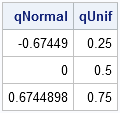Notice that sample quantiles of data are computed by using the QNTL call, but theoretical quantiles of a "named" distribution are computed by using the QUANTILE function in Base SAS.

### Computing other quantiles

The QNTL call supports a third parameter with which you can specify specific quantiles. For example, the following statements compute the 5th, 10th, 90th, and 95th quantiles of each column of x:

```p = {0.05, 0.10, 0.90, 0.95}; call qntl(q, x, p); /* compute 5th, 10th, 90th, and 95th quantiles */```

### Labeling quantiles

The output from the QNTL call is a matrix, q. The number of columns of q is the number of n columns of x, and each row represents a quantile. If you want to display the quantiles, it is convenient to use the CHAR function or the PUTN function to form row labels that indicate the quantiles or percentiles, as shown in the following statements:

```labels = "P" + putn(100*p, "Z2."); /* concat "P" and {"05" "10" "90" "95"} */ varNames = {"Normal" "Uniform"}; print q[rowname=labels colname=VarNames];```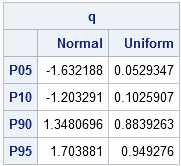As shown in the example, the Zw.d format can be useful for converting the numerical quantiles to percentile labels.I work with continuous distributions more often than with discrete distributions. Consequently, I am used to thinking of the quantile function as being an inverse cumulative distribution function (CDF). (These functions are described in my article, "Four essential functions for statistical programmers.")

For discrete distributions, they are not. To quote from my "Four essential functions" article: "For discrete distributions, the quantile is the smallest value for which the CDF is greater than or equal to the given probability." (Emphasis added.)

There is a simple numerical way to examine the relationship between the quantile and CDF: call one function after the other and see if the resulting answer is the same value that you started with. (In other words, compose the functions to see if they are the identity function.) The following SAS/IML statements compute a normal quantile, followed by a CDF:

```proc iml; /* the quantile function is the inverse CDF for continuous distributions */ prob = 0.8; /* start with 0.8 */ q = quantile("Normal", prob); /* compute normal quantile z_0.8 */ cdf = cdf("Normal", q); /* compute CDF(z_0.8) */ print prob q cdf; /* get back to 0.8 */```As expected, the QUANTILE function and the CDF function are inverse operations for a continuous distribution such as the normal distribution. However, this is not true for discrete distributions such as the binomial distribution:

```/* the quantile function is NOT the inverse CDF for discrete distributions */ prob = 0.8; q = quantile("Binomial", prob, 0.5, 10); /* q = 80th pctl of Binom(p,n) */ cdf = cdf("Binomial", q, 0.5, 10); /* CDF(q) does NOT equal 0.8 */ print prob q cdf;```The reason becomes apparent by looking at the CDF function for the binomial distribution. Consider 10 tosses of a fair coin that has probability p=0.5 of landing on "heads." The Binom(0.5, 10) distribution models this experiment. The CDF function displays the probability that the ten tosses will result in m heads, for m=0, 1, ..., 10, as shown in the following graph:

```data BinomCDF(drop=p N); p = 0.5; N = 10; do m = 0 to N by 0.1; cdf = cdf("Binomial", m, p, N); output; end; run;   proc sgplot data=BinomCDF; title "Probability of m heads in 10 coin tosses"; title2 "CDF of Binom(0.5, 10)"; scatter x=m y=cdf; xaxis label="Number of Heads" values=(0 to 10) grid; yaxis label="P(Number of heads <= m)" grid; run;```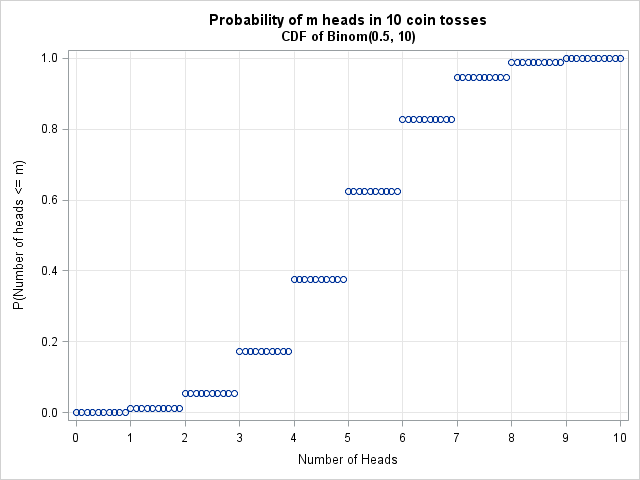The CDF function is a step function that maps an entire interval to a single probability. For example, the entire interval [5, 6) is mapped to the value 0.623. The quantile function looks similar and maps intervals to the integers 0, 1, ..., 9, 10. For example, the binomial quantile of x is 5 for every x in the interval (0.377, 0.623). This example generalizes: the quantile for a discrete distribution always returns a discrete value.

A consequence of this fact was featured in my article on "Funnel plots for proportions." Step 3 of creating a funnel plot is complicated because it computes a continuous approximation to discrete control limits that arise from binomial quantiles. If you approximate the binomial distribution by a normal distribution, Step 3 becomes simpler to implement, but the funnel curves based on normal quantiles are different from the curves based on binomial quantiles. A future article will explore how well the normal quantiles approximate binomial quantiles.As a SAS developer, I am always looking ahead to the next release of SAS. However, many SAS customer sites migrate to new releases slowly and are just now adopting versions of SAS that were released in 2010 or 2011. Consequently, I want to write a few articles that discuss recent additions to the SAS/IML language, where "recent" goes back a few years. For the several Mondays, my "Getting Started" articles will review SAS/IML language features that were added in SAS/IML 9.22 (released in 2010) and SAS/IML 9.3 (released in 2011).

Today's topic: basic descriptive statistics for sample data. In particular, the MEAN, VAR, and STD functions.

### The MEAN function: Much more than sample means

Prior to SAS/IML 9.22, statistical programmers used the colon (:) subscript reduction operator to compute the arithmetic mean of data. For example, the following SAS/IML program computes the grand mean, the row means, and the column means of data in a 5x2 matrix:

```proc iml; x = {-1 -1, 0 1, 1 2, 1 0, -1 0 };   rowMeans = x[ ,:]; colMeans = x[:, ]; grandMean= x[:]; print x rowMeans, colMeans grandMean;```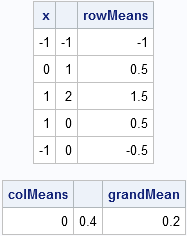The MEAN function was introduced in SAS/IML 9.22. The expression mean(x) computes the arithmetic mean of each column of a matrix. It is equivalent to x[:,]. The MEAN function also supports trimmed and Winsorized means, which are robust estimators of location.

Because the MEAN function computes the arithmetic mean of each column of a matrix, you need to be careful when computing the mean of a vector. Make sure that the function argument it is a column vector, not a row vector. For example, the following statement does NOT compute the mean of the elements in the vector, g:

```g = 1:5; /* row vector {1 2 3 4 5} */ m = mean(g); /* probably not what you want! */```

Instead, use the transpose function (T) or the COLVEC function so that the argument to the MEAN function is a column vector:

`m = mean(colvec(g)); /* correct */`

A previous article discusses the trimmed and Winsorized means and provides an example.

### The VAR function for computing the sample variance

Prior to SAS/IML 9.22, statistical programmers could use a module to compute the sample variance of each column of a matrix. The VAR function is more efficient, but the results are the same. The following statement computes the sample variance of each column of x:

```v = var(x); print v;```If you compute the variance of data in a vector, make sure that you pass a column vector to the VAR function.

### The STD function for computing the sample standard deviation

The STD function (introduced in SAS 9.3) is simply the square root of the sample variance. As such, the STD function is merely a convenient shortcut for sqrt(var(x)):

```s = std(x); print s;```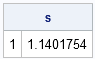Once again, if you compute the standard deviation of data in a vector, make sure that you pass a column vector to the STD function.I've blogged several times about multivariate normality, including how to generate random values from a multivariate normal distribution. But given a set of multivariate data, how can you determine if it is likely to have come from a multivariate normal distribution?

The answer, of course, is to run a goodness-of-fit (GOF) test to compare properties of the data with theoretical properties of the multivariate normal (MVN) distribution. For univariate data, I've written about the usefulness of the quantile-quantile (Q-Q) plot to model the distribution of data, and it turns out that there is a similar plot that you can use to assess multivariate normality. There are also analytic GOF tests that can be used.

To see how these methods work in SAS, we need data. Use the RANDNORMAL function in SAS/IML software to generate data that DOES come from a MVN distribution, and use any data that appears nonnormal to examine the alternative case. For this article, I'll simulate data that is uniformly distributed in each variable to serve as data that is obviously not normal. The following SAS/IML program simulates the data:

```proc iml; N = 100; /* 100 obs for each distribution */ call randseed(1234);   /* multivariate normal data */ mu = {1 2 3}; Sigma = {9 1 2, 1 6 0, 2 0 4 }; X = randnormal(N, mu, Sigma);   /* multivariate uniform data */ v = j(N, ncol(mu)); /* allocate Nx3 matrix*/ call randgen(v, "Uniform"); /* each var is U[0,1] */ v = sqrt(12)*(v - 1/2); /* scale to mean 0 and unit variance */ U = mu + T(sqrt(vecdiag(Sigma))) # v; /* same mean and var as X */```

### A graphical test of multivariate normality

If you want a quick check to determine whether data "looks like" it came from a MVN distribution, create a plot of the squared Mahalanobis distances versus quantiles of the chi-square distribution with p degrees of freedom, where p is the number of variables in the data. (For our data, p=3.) As I mentioned in the article on detecting outliers in multivariate data, the squared Mahalanobis distance has an approximate chi-squared distribution when the data are MVN. See the article "What is Mahalanobis distance?" for an explanation of Mahalanobis distance and its geometric interpretation.

I will use a SAS/IML function that computes Mahalanobis distances. You can insert the function definition into the program, or you can load the module from a SAS catalog if it was previously stored. The following program computes the Mahalanobis distance between the rows of X and the sample mean:

```load module=Mahalanobis; /* or insert module definition here */   Mean = mean(X); /* compute sample mean and covariance */ Cov = cov(X); md = mahalanobis(X, Mean, Cov);```

For MVN data, the square of the Mahalanobis distance is asymptotically distributed as a chi-square with three degrees of freedom. (Note: for a large number of variables you need a very large sample size before the asymptotic chi-square behavior becomes evident.) To plot these quantities against each other, I use the same formula that PROC UNIVARIATE uses to construct its Q-Q plots, as follows:

```md2 = md##2; call sort(md2, 1); s = (T(1:N) - 0.375) / (N + 0.25); chisqQuant = quantile("ChiSquare", s, ncol(X));```

If you plot md2 versus chiSqQuant, you get the graph on the left side of the following image. Because the points in the plot tend to fall along a straight line, the plot suggests that the data are distributed as MVN. In contrast, the plot on the right shows the same computations and plot for the uniformly distributed data. These points do not fall on a line, indicating that the data are probably not MVN. Because the samples contain a small number of points (100 for this example), you should not expect a "perfect fit" even if the data are truly distributed as MVN.### Goodness-of-fit tests for multivariate normality

Mardia's (1974) test multivariate normality is a popular GOF test for multivariate normality. Mardia (1970) proposed two tests that are based definitions of multivariate skewness and kurtosis. (See von Eye and Bogat (2004) for an overview of this and other methods.) It is easy to implement these tests in the SAS/IML language.

However, rather than do that, I want to point out that SAS provides the %MULTNORM macro that implements Mardia's tests. The macro also plots the squared Mahalanobis distances of the observations to the mean vector against quantiles of a chi-square distribution. (However, it uses the older GPLOT procedure instead of the newer SGPLOT procedure.) The macro requires either SAS/ETS software or SAS/IML software. The following statements define the macro and call it on the simulated MVN data:

```/* write data from SAS/IML to SAS data set */ varNames = "x1":"x3"; create Normal from X[c=varNames]; append from X; close Normal; quit;   /* Tests for MV normality */ %inc "C:\path of macro\multnorm.sas"; %multnorm(data=Normal, var=x1 x2 x3, plot=MULT);```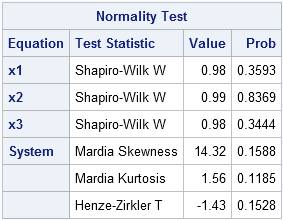The macro generates several tables and graphs that are not shown here. The test results shown in the preceding table indicate that there is no reason to reject the hypothesis that the sample comes from a multivariate normal distribution. In addition to Mardia's test of skewness and kurtosis, the macro also performs univariate tests of normality on each variable and another test called the Henze-Zirkler test.

### Another graphical tool: Plot of marginal distributions

To convince yourself that the simulated data are multivariate normal, it is a good idea to use the SGSCATTER procedure to create a plot of the univariate distribution for each variable and the bivariate distribution for each pair of variables. Alternatively, you can use the CORR procedure as is shown in the following statements. The CORR procedure can also produce the sample mean and sample covariance, but these tables are not shown here.

```/* create scatter plot matrix of simulated data */ proc corr data=Normal COV plots(maxpoints=NONE)=matrix(histogram); var x:; ods select MatrixPlot; run;```The scatter plot matrix shows (on the diagonal) that each variable is approximately normally distributed. The off-diagonal elements show that the pairwise distributions are bivariate normal. This is characteristic of multivariate normal data: all marginal distributions are also normal. (This explains why the %MULTNORM macro includes univariate tests of normality in its test results.) Consequently, the scatter plot matrix is a useful graphical tool for investigating multivariate normality.I previously described how to use Mahalanobis distance to find outliers in multivariate data. This article takes a closer look at Mahalanobis distance. A subsequent article will describe how you can compute Mahalanobis distance.

### Distance in standard units

In statistics, we sometimes measure "nearness" or "farness" in terms of the scale of the data. Often "scale" means "standard deviation." For univariate data, we say that an observation that is one standard deviation from the mean is closer to the mean than an observation that is three standard deviations away. (You can also specify the distance between two observations by specifying how many standard deviations apart they are.)

For many distributions, such as the normal distribution, this choice of scale also makes a statement about probability. Specifically, it is more likely to observe an observation that is about one standard deviation from the mean than it is to observe one that is several standard deviations away. Why? Because the probability density function is higher near the mean and nearly zero as you move many standard deviations away.

For normally distributed data, you can specify the distance from the mean by computing the so-called z-score. For a value x, the z-score of x is the quantity z = (x-μ)/σ, where μ is the population mean and σ is the population standard deviation. This is a dimensionless quantity that you can interpret as the number of standard deviations that x is from the mean.

### Distance is not always what it seems

You can generalize these ideas to the multivariate normal distribution. The following graph shows simulated bivariate normal data that is overlaid with prediction ellipses. The ellipses in the graph are the 10% (innermost), 20%, ..., and 90% (outermost) prediction ellipses for the bivariate normal distribution that generated the data. The prediction ellipses are contours of the bivariate normal density function. The probability density is high for ellipses near the origin, such as the 10% prediction ellipse. The density is low for ellipses are further away, such as the 90% prediction ellipse.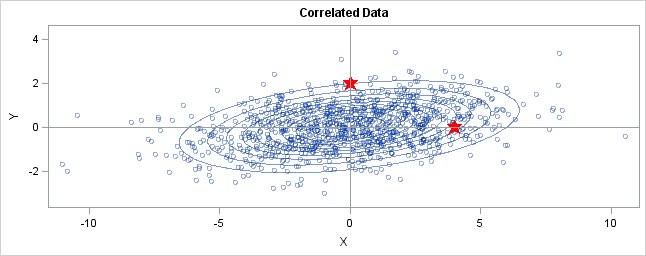In the graph, two observations are displayed by using red stars as markers. The first observation is at the coordinates (4,0), whereas the second is at (0,2). The question is: which marker is closer to the origin? (The origin is the multivariate center of this distribution.)

The answer is, "It depends how you measure distance." The Euclidean distances are 4 and 2, respectively, so you might conclude that the point at (0,2) is closer to the origin. However, for this distribution, the variance in the Y direction is less than the variance in the X direction, so in some sense the point (0,2) is "more standard deviations" away from the origin than (4,0) is. </p

Notice the position of the two observations relative to the ellipses. The point (0,2) is located at the 90% prediction ellipse, whereas the point at (4,0) is located at about the 75% prediction ellipse. What does this mean? It means that the point at (4,0) is "closer" to the origin in the sense that you are more likely to observe an observation near (4,0) than to observe one near (0,2). The probability density is higher near (4,0) than it is near (0,2).

In this sense, prediction ellipses are a multivariate generalization of "units of standard deviation." You can use the bivariate probability contours to compare distances to the bivariate mean. A point p is closer than a point q if the contour that contains p is nested within the contour that contains q.

### Defining the Mahalanobis distance

You can use the probability contours to define the Mahalanobis distance. The Mahalanobis distance has the following properties:

• It accounts for the fact that the variances in each direction are different.
• It accounts for the covariance between variables.
• It reduces to the familiar Euclidean distance for uncorrelated variables with unit variance.

For univariate normal data, the univariate z-score standardizes the distribution (so that it has mean 0 and unit variance) and gives a dimensionless quantity that specifies the distance from an observation to the mean in terms of the scale of the data. For multivariate normal data with mean μ and covariance matrix Σ, you can decorrelate the variables and standardize the distribution by applying the Cholesky transformation z = L-1(x - μ), where L is the Cholesky factor of Σ, Σ=LLT.

After transforming the data, you can compute the standard Euclidian distance from the point z to the origin. In order to get rid of square roots, I'll compute the square of the Euclidean distance, which is dist2(z,0) = zTz. This measures how far from the origin a point is, and it is the multivariate generalization of a z-score.

You can rewrite zTz in terms of the original correlated variables. The squared distance Mahal2(x,μ) is
= zT z
= (L-1(x - μ))T (L-1(x - μ))
= (x - μ)T (LLT)-1 (x - μ)
= (x - μ)T Σ -1 (x - μ)
The last formula is the definition of the squared Mahalanobis distance. The derivation uses several matrix identities such as (AB)T = BTAT, (AB)-1 = B-1A-1, and (A-1)T = (AT)-1. Notice that if Σ is the identity matrix, then the Mahalanobis distance reduces to the standard Euclidean distance between x and μ.

The Mahalanobis distance accounts for the variance of each variable and the covariance between variables. Geometrically, it does this by transforming the data into standardized uncorrelated data and computing the ordinary Euclidean distance for the transformed data. In this way, the Mahalanobis distance is like a univariate z-score: it provides a way to measure distances that takes into account the scale of the data.In two previous blog posts I worked through examples in the survey article, "Robust statistics for outlier detection," by Peter Rousseeuw and Mia Hubert. Robust estimates of location in a univariate setting are well-known, with the median statistic being the classical example. Robust estimates of scale are less well-known, with the best known example being interquartile range (IQR), but a more modern statistic being the MAD function.

For multivariate data, the classical (nonrobust) estimate of location is the vector mean, c, which is simply the vector whose ith component is the mean of the ith variable. The classical (nonrobust) estimate of scatter is the covariance matrix. An outlier is defined as an observation whose Mahalanobis distance from c is greater than some cutoff value. As in the univariate case, both classical estimators are sensitive to outliers in the data. Consequently, statisticians have created robust estimates of the center and the scatter (covariance) matrix.

## MCD: Robust estimation by subsampling

A popular algorithm that computes a robust center and scatter of multivariate data is known as the Minimum Covariance Determinant (MCD) algorithm. The main idea is due to Rousseeuw (1985), but the algorithm that is commonly used was developed by Rousseeuw and Van Driessen (1999). The MCD algorithm works by sampling h observations from the data over and over again, where h is typically in the range n/2 < h < 3n/4. The "winning" subset is the h points whose covariance matrix has the smallest determinant. Points far from the center of this subset are excluded, and the center and scatter of the remaining points are used as the robust estimates of location and scatter.

This Monte Carlo approach works very well in practice, but it does have the unfortunate property that it is not deterministic: a different random number seed could result in different robust estimates. Recently, Hubert, Rousseeuw, and Verdonck (2010) have published a deterministic algorithm for the MCD.

## Robust MCD estimates in SAS/IML software

The SAS/IML language includes the MCD function for robust estimation of multivariate location and scatter. The following matrix defines a data matrix from Brownlee (1965) that correspond to certain measurements taken on 21 consecutive days. The points are shown in a three-dimensional scatter plot that was created in SAS/IML Studio.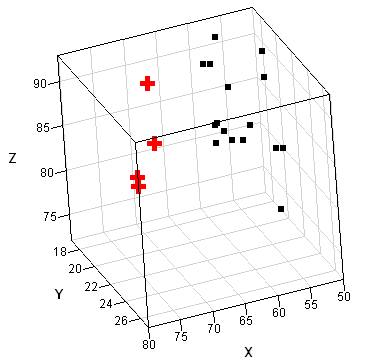```proc iml; x = { 80 27 89, 80 27 88, 75 25 90, 62 24 87, 62 22 87, 62 23 87, 62 24 93, 62 24 93, 58 23 87, 58 18 80, 58 18 89, 58 17 88, 58 18 82, 58 19 93, 50 18 89, 50 18 86, 50 19 72, 50 19 79, 50 20 80, 56 20 82, 70 20 91 };   /* classical estimates */ labl = {"x1" "x2" "x3"}; mean = mean(x); cov = cov(x); print mean[c=labl format=5.2], cov[r=labl c=labl format=5.2];```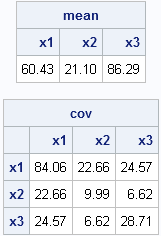Most researchers think that observations 1, 2, 3, and 21 are outliers, with others including observation 2 as an outlier. (These points are shown as red crosses in the scatter plot.) The following statement runs the MCD algorithm on these data and prints the robust estimates:

```/* robust estimates */ N = nrow(x); /* 21 observations */ p = ncol(x); /* 3 variables */   optn = j(8,1,.); /* default options for MCD */ optn = 0; /* =1 if you want printed output */ optn= floor(0.75*N); /* h = 75% of obs */   call MCD(sc, est, dist, optn, x); RobustLoc = est[1, ]; /* robust location */ RobustCov = est[3:2+p, ]; /* robust scatter matrix */ print RobustLoc[c=labl format=5.2], RobustCov[r=labl c=labl format=5.2];```The robust estimate of the center of the data is not too different from the classical estimate, but the robust scatter matrix is VERY different from the classical covariance matrix. Each robust estimate excludes points that are identified as outliers.

If you take these robust estimates and plug them into the classical Mahalanobis distance formula, the corresponding distance is known as the robust distance. It measures the distance between each observation and the estimate of the robust center by using a metric that depends on the robust scatter matrix. The MCD subroutine returns distance information in a matrix that I've called DIST (the third argument). The first row of DIST is the classical Mahalanobis distance. The second row is the robust distance, which is based on the robust estimates of location and scatter. The third row is an indicator variable with the value 1 if an observation is closer to the robust center than some cutoff value, and 0 otherwise. Consequently, the following statements find the outliers.
```/* rules to detect outliers */ outIdx = loc(dist[3,]=0); /* RD > cutoff */ print outIdx;```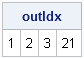The MCD algorithm has determined that observations 1, 2, 3, and 21 are outliers.

Incidentally, the cutoff value used by MCD is based on a quantile of the chi-square distribution because the squared Mahalanobis distance of multivariate normal data obeys a chi-square distribution with p degress of freedom, where p is the number of variables. The cutoff used is as follows:
`cutoff = sqrt( quantile("chisquare", 0.975, p) ); /* dist^2 ~ chi-square */`

In a recent paper, Hardin and Rocke (2005) propose a different criterion, based on the distribution of robust distances.

## Robust MCD estimates in SAS/STAT software: How to "trick" PROC ROBUSTREG

The ROBUSTREG procedure can also compute MCD estimates. Usually, the ROBUSTREG procedure is used as a regression procedure, but you can also use it to obtain the MCD estimates by "inventing" a response variable. The MCD estimates are produced for the explanatory variables, so the choice of a response variable is unimportant. In the following example, I generate random values for the response variable.

In a regression context, the word "outlier" is reserved for an observation for which the value of the response variable is far from the predicted value. In other words, in regression an outlier means "far away (from the model) in the Y direction." In contrast, the ROBUSTREG procedure uses the MCD algorithm to identify influential observations in the space of explanatory (that is, X) variables. These are also called high-leverage points. They are observations that are far from the center of the X variables. High-leverage points are very influential in ordinary least squares regression, and that is why it is important to identify them.

To generate the MCD estimates, specify the DIAGNOSTICS and the LEVERAGE(MCDINFO) options on the MODEL statement, as shown in the following statements:

```/* write data from SAS/IML (or use a DATA step) */ create X from x[c=labl]; append from x; close; quit;   data X; set X; y=rannor(1); /* random response variable */ run;   proc robustreg data=X method=lts; model y = x1 x2 x3 / diagnostics leverage(MCDInfo); ods select MCDCenter MCDCov Diagnostics; ods output diagnostics=Diagnostics(where=(leverage=1)); run;   proc print data=Diagnostics; var Obs Mahalanobis RobustDist Leverage; run;```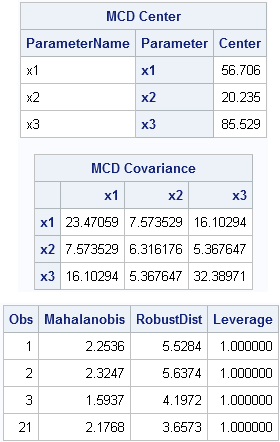The robust estimates of location and scatter are the same, as are the robust distances. The "leverage" variable is an indicator variable that tells you which observations are far from the center of the explanatory variables. They are multivariate "outliers" in the the space of the X variables, although they are not necessarily outliers for the response (Y) variable.In a previous blog post on robust estimation of location, I worked through some of the examples in the survey article, "Robust statistics for outlier detection," by Peter Rousseeuw and Mia Hubert. I showed that SAS/IML software and PROC UNIVARIATE both support the robust estimators of location that are mentioned in the paper. Today's post looks at the robust estimators of scale that are mentioned in the same paper and works through more examples in the paper. The paper uses the following five measurements, which contain one outlier:
6.25, 6.27, 6.28, 6.34, 63.1

## Robust scale statistics in SAS/IML software

SAS/IML software contains several functions for robust estimation of scale. For estimating scale, the MAD function is often used. The MAD statistic is an acronym for "median of all absolute deviations from the median." The MAD statistic is often multiplied by a constant in order to make it unbiased for data that are normally distributed. The constant is 1.483, but you don't need to remember that value because the MAD function has the "NMAD" option that automatically includes the multiplication factor, as shown in the following example:

```proc iml; x = {6.25, 6.27, 6.28, 6.34, 63.1}; mad = mad(x, "NMAD"); print mad;```Rousseeuw and Hubert briefly mention two other robust measures of scale: the Qn estimator (Rousseeuw and Croux, JASA, 1993) and the interquartile range (IQR), which is well-known from the Tukey box plot. You can compute both of these estimators in SAS/IML software, as follow:

```Qn = mad(x, "QN"); call qntl(q, x, {0.25 0.75}); /* compute 25th and 75th percentile */ IQR = q - q; print Qn IQR;```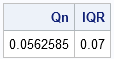The three robust estimates of scale are similar. They range from 0.04 (MAD) to 0.07 (IQR). The IQR is sometimes divided by 1.349 in order to estimate the scale of normally distributed data. If you divide 0.07 by 1.349, you get 0.052, which make the estimates even more similar.

## The connection with outlier detection

All this discussion of robust estimation of location and scale is closely related to detecting outliers. In practice, outliers are often detected using a rule or formula. The classical rule is to compute z-scores, which are just the normalized values zi = (xi - x̄)/s, where is the sample mean and s is the sample standard deviation. An outlier is defined as any observation for which |zi| exceeds some cutoff value, typically 2.5 or 3.

This rule fails when there is a large outlier in the data. For example, the following SAS/IML statements compute the classical z-scores for the Rousseeuw and Hubert example:

```/* rules to detect outliers */ z = (x - mean(x)) / std(x); print z;```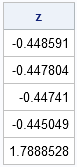Because the mean and standard deviation are both influenced by the outlier, no observation has a large z-score, and therefore none is flagged as an outlier. However, using robust estimators in the z-score formula does successfully identify the outlier, as shown in the following statements:

```zRobust = (x - median(x)) / mad(x, "NMAD"); print zRobust;```The outlier has a HUGE "robust score." Of course, you don't have to print out the scores and inspect them. The following SAS/IML statements use the LOC function (the most useful function that you've never heard of!) to find all of the data for which the robust z-score exceeds 2.5, and prints only the outliers:

```outIdx = loc(abs(zRobust)>2.5); if ncol(outIdx)>0 then outliers = x[outIdx]; else outliers = .; print outliers;```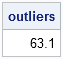## Robust Estimates in the UNIVARIATE Procedure

The UNIVARIATE procedure also supports robust estimates of scale. The ROBUSTSCALE option on the PROC UNIVARIATE statement computes the robust estimates in the Rousseeuw and Hubert article, as well as others. The documentation for the UNIVARIATE procedure includes a section that describes the robust estimates of scale. The following example computes robust estimates of scale:

```data a; input x @@; datalines; 6.25 6.27 6.28 6.34 63.1 ; run;   proc univariate data=a robustscale; var x; ods select RobustScale; run;```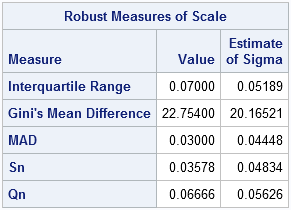Notice that the output from PROC UNIVARIATE includes two columns. The first column is an unadjusted robust estimate. The second column estimates the standard deviation for normally distributed data, which can be derived from the first column.